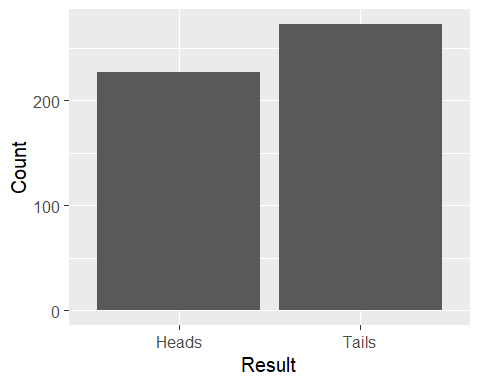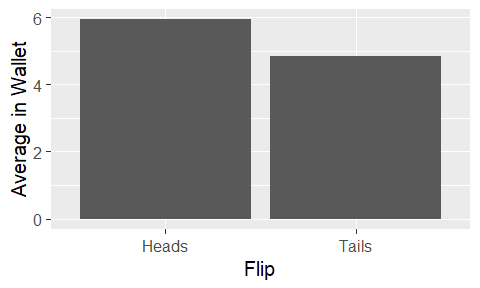# Lecture 2: Understanding Data

## What’s the Point?

What are we actually trying to DO when we use data?

Contrary to popular opinion, the point isn’t to make pretty graphs or to make a point, or justify something you’ve done.

Those may be nice side effects!

## Uncovering Truths

The cleanest way to think about data analysis is to remember that data comes from somewhere

There was some process that generated that data

Our goal, in all data analysis, is to get some idea of what that process is

## Example

• Imagine a basic coin flip
• Every time we flip, we get heads half the time and tails half the time
• The TRUE process that generates the data is that there’s a coin that’s heads half the time and tails half the time
• If we analyze the data correctly, we should report back that the coin is heads half the time
• Let’s try calculating the proportion of heads

## Example

``````#Generate 500 heads and tails
``##  0.454``## Example

• Let’s try out that code in R a few times and see what happens
• First, what do we want to happen? What should we see if our data analysis method is good?

## How Good Was It?

• Our data analysis consistently told us that the coin was generating heads about half the time - the true process!
• Our data analysis lets us conclude the coin is fair
• That is describing the true data generating process pretty well!
• Let’s think - what other approaches could we have taken? What would the pros and cons be?
• Taking the mean and adding .1?
• Just saying it’s 50%?

## Another Example

• People have different amounts of money in their wallet, from 0 to 10
• We flip a coin and, if it’s heads, give them a dollar
• What’s the data generating process here?
• What should our data analysis uncover?

## Another Example

``````#Generate 1000 wallets and 1000 heads and tails
data <- data.frame(wallets=sample(0:10,1000,replace=TRUE))
#Give a dollar whenever it's a heads, then get average money by coin
data <- data %>% mutate(wallets = wallets + (coin=="Heads"))
data %>% group_by(coin) %>% summarize(wallets = mean(wallets))``````
``````## # A tibble: 2 x 2
##   coin  wallets
##   <chr>   <dbl>
## 2 Tails    4.84``````## Conclusions

• What does our data analysis tell us?
• We observe a difference of 1.09 between the heads and tails
• Because we know that nothing would have caused this difference other than the coin flip, we can conclude that the coin flip is why there’s a difference of 1.09

## But What If?

• So far we’ve been cheating
• We know exactly what process generated that data
• So really, our data analysis doesn’t matter
• But what if we don’t know that?
• We want to make sure our method is good, so that when we draw a conclusion from our data, it’s the right one

## Example 3

• We’re economists! So no big surprise we might be interested in demand curves
• Demand curve says that as \(P\) goes up, \(Q_d\) goes down
• Let’s gather data on \(P\) and \(Q_d\)
• And determine things like the slope of demand, demand elasticity, etc.
• Just so happens I have some data on 1879-1923 US food export prices and quantities!